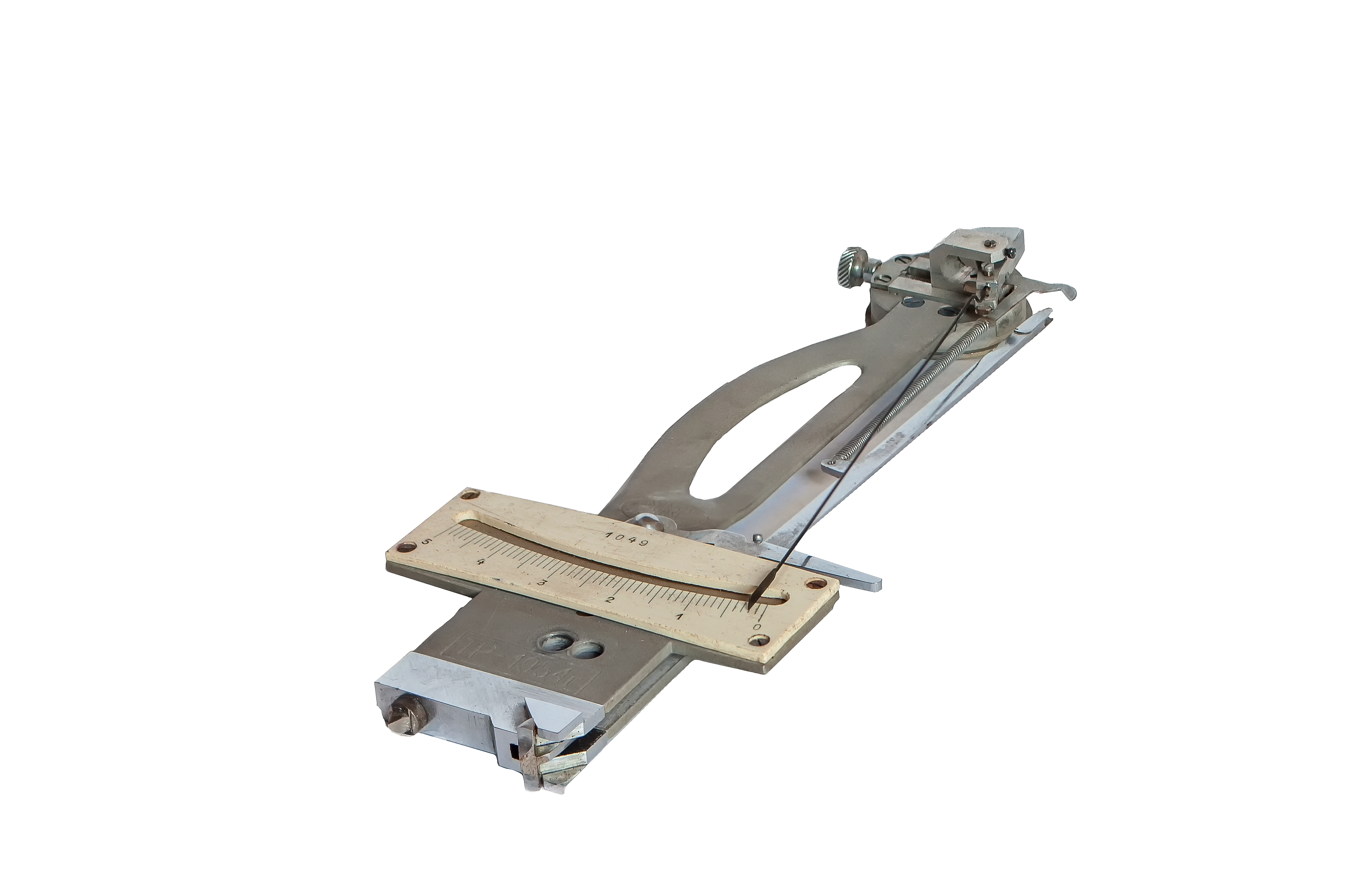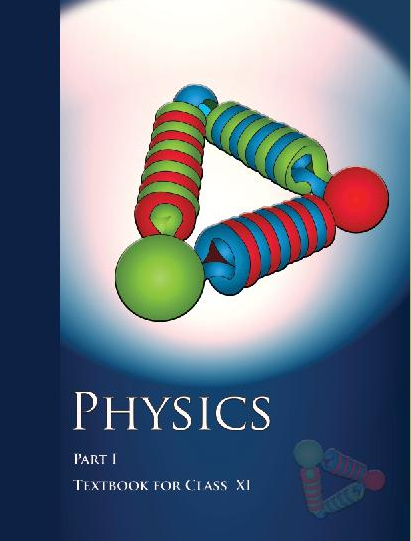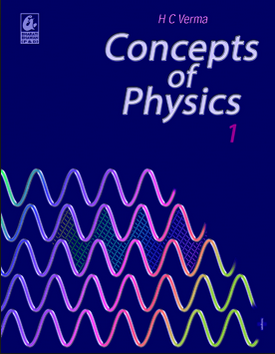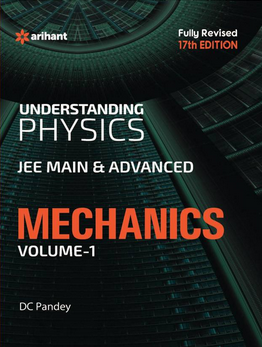# Experimental skills   Share

## What is Experimental skills

Science is all about experiments, as all the hypothesis can become a law only when they are justified experimentally. Experimentation involves lots of terms like precision and accuracy etc. also, all the observations and their corresponding values obtained are approximate. However, how much approximation we have to consider depends upon the apparatus used. In this unit, we will explore and find out all about errors and then move on to another powerful tool named as dimensional analysis. While closing the topic we will look at a few experiments, which are used to determine some physical quantities.

Experimental skills provide us with knowledge of the physical world, and it is the experiments that provide us the evidence that grounds this knowledge. One of the major roles of experimental skills is to test theories and to provide the basis for scientific knowledge.

Let us take an example of measuring the diameter of ball bearings of a bicycle. As we know that the diameter of the ball bearing is very small. Even a small error during the measurement of the diameter of the ball can make the unbalancing of the bicycle.Let us take an example that we want to hang a ceiling fan. The rod which is used to hang the fan should have enough strength to take the weight of the fan. So we need to calculate Young's modulus of elasticity of the material. If Young's modulus of elasticity of the material is lower than required, the fan will fall down.From the exam point of view, it plays a vital role while scoring much better. So apart from the practical application, this is very much important in the entrance as well as school examinations.

## Notes on Experimental Skills-

So we will discuss step by step about important topics, procedure and results from this chapter followed by an overview of this chapter. Then we will understand important formulas and experiments from this chapter. Remembering these formulae, concepts, and procedure will increase your speed while question-solving and experimental skills. Now let us take a look on topics and overview of this particular chapter.

Prepare Online for JEE Main/NEET

Crack JEE 2021 with JEE/NEET Online Preparation Program

## Experimental Skills Topics

• Vernier calipers, its use to measure external and internal diameter and depth of a given vessel.

• Screw gauge and its use to determine the diameter/thickness of thin sheet/wire.

• Simple Pendulum-All the dissipation of energy by plotting a graph between square of amplitude versus time.

• Metre Scale: Calculate the mass of the given object by principle of moments.

• Young's modulus of elasticity of the material used in the metallic wire.

• The surface tension of water by using capillary rise and effect of detergents on it.

• Calculation of Coefficient of the viscosity of a given viscous liquid by measuring the terminal velocity of a spherical body.

• Plotting a cooling curve between the temperature of a hot body versus time.

• Calculate the speed of sound in air at room temperature using a resonance tube.

• Specific heat capacity of a given (i) solid and (ii) liquid by method of mixtures.

• Find the resistivity of the material of a given wire using metre bridge.

• Calculate the resistance of a given wire using Ohm's law.

• Potentiometer-

a) Comparison between emf of two primary cells.

b) Determination of the internal resistance of the given cell.

• Calculate the resistance and figure of merit of a galvanometer by half deflection method,

• The focal length of the optical equipment by using the parallax method:

a) Convex mirror

b) Concave mirror, and

c) Convex lens

• Plot the graph between angle of deviation vs angle of incidence for a given triangular prism.

• Calculate the refractive index of a glass slab using a traveling microscope.

• Characteristic curves of a p-n junction diode in reverse and forward bias.

• Characteristic curves of  Zener diode and finding reverse break-down voltage.

• Characteristic curves of the transistor and finding current gain and voltage gain.

• Identification of Diodes, Transistor, LED, IC, Capacitor, Resistor from mixed collection of such items.

• Using a multimeter to:

1. Identify the base of a transistor.
2. Distinguish between n-p-n and p-n-p  type transistor
3. See the unidirectional flow of current in case of a LED and a Diode.
4. Check the correctness or otherwise of a given electronic component (Transistor, Diode or IC).

## Experimental Skills Overview

• The Vernier caliper is a measuring instrument used to measure dimensions very precisely. It is used to measure external and internal lengths accurately, the error may be as little as 0.05 mm, depending on the caliper.

• Screw gauge is a device in which there is a calibrated screw. It is widely used for accurate measurement of equipment in mechanical engineering and machining.

• When the bob of a simple pendulum oscillates, its amplitude gets decreased and hence its energy will also decrease. The energy gets dissipated due to the damping force (such as air resistance etc.) experienced by the bob of the pendulum. So we will draw a graph between the square of amplitude versus time.

• In this experiment of meter scale - you will use the principle of moments, together with the idea of the centre of gravity, to find the mass of a metre rule.

• Young’s modulus determination is very important for the determination of the strength of the material. We will calculate the strain in this experiment.

• The surface tension of different detergent solutions (colloidal) in water will be compared. In a detergent solution, the capillary rise (or the surface tension) will be lower than that for pure and distilled water.

• When a spherical body moves down through an infinite column of viscous liquid, it drags the layer of the liquid in contact with it. So we will use Stoke’s law for calculation of Co-efficient of the viscosity of a given viscous liquid by measuring the terminal velocity of a spherical body.

• The temperature is seen to fall quickly in the beginning and then the difference in temperature slowly decreases. So it decreases exponentially. It can be deduced by Newton’s law of cooling.

• We use the resonance tube to find the speed of sound in air. As the velocity of sound in air is to be found by using tuning forks of known frequency. By making use of the resonance of an air column, the wavelength of the sound will be determined.

• The measurement of heat quantities is carried out by what is known as the method of mixtures;  this will makes use of the principle that when a heat exchange takes place between two bodies initially at different temperatures, the quantity of heat lost by the warmer body is equal to that gained by cooler body, and some intermediate equilibrium temperature is finally reached.  This is true when it is provided that no heat is gained from or lost to the surroundings.

• To find the resistivity we use the concept of meter bridge and the concept of Wheatstone’s network.

• By using Ohm’s law for given conditions, we will find the resistance of the given material.

• Potentiometer - It is a three-terminal resistor which contains a rotating or sliding contact which forms an adjustable voltage divider. By use of this we will find the a) Comparison between emf of two primary cells b) Determination of the internal resistance of a given cell.

• Parallax- It is defined as a displacement in the apparent position of an object which is viewed along two different lines of sight, and is measured by the angle or semi-angle of inclination between those two lines. So by using this method we will find the focal length of the different kind of optical devices.

• We know that the angle of deviation depends upon the angle of incidence. So we will go through the experiments to Plot the graph between angle of deviation vs angle of incidence for a triangular prism.

• For p-n junction diode, we will draw the graph for forward and reverse bias.

• For Zener diode, we will draw the characteristics curve of this and reverse break-down voltage.

• For the transistor, we will draw the characteristics curve and then calculate the current and voltage gain.

• Identification of Diodes, Transistor, LED, IC, Capacitor, Resistor from mixed collection of such items.

• A multimeter is an electronic measuring device that combines several measurement functions in one particular unit. A typical multimeter can measure voltage, current, and resistance in a single unit. So we will use it for - a) Identify the base of a transistor.b) Distinguish between n-p-n and p-n-p type transistor c) See the unidirectional flow of current in case of a LED and an Diode. d) Check the correctness or otherwise of a given electronic component (Transistor, Diode or IC).

## How to prepare Experimental skills

First, you should have good command over the basic theory of all the experiments and you should also know how to apply them well at the time of the experiments or while making table and observations. Please try to understand each concept from this chapter, with the help of theory, experiments, questions and video lectures on each important concept. For each concept practice enough problems so that you have a thorough understanding of the concept. Do all the experiments at your lab and solve all the questions at home with proper concentration and try to do all observation table by yourself.

How to do Experiments -

1. Make observations

2. Form a hypothesis

3. Make a prediction.

4. Perform an experiment.

5. Analyze the results of the experiment.

6. Draw a conclusion.

Develop skills to do experiments by practicing more. This will also help to get more interest in real Physics. You will come to know about a lot about Physics and the real-world scenario of the use of Physics. Experiments make your Physics concepts more clear.

## Experimental skills Tips

1. This chapter is more practical based chapter there is very little to memorize, hence practice is the key here for getting a good hold on this chapter.

2. Do the experiments regularly and match with the existing data. If you are always coming close to authorized result then only proceed further.

3. Always follow the full procedure and never take shortcuts to avoid errors.

4. Make a plan to prepare for this chapter and Stick to a Timetable.

5. Use the manuals and authorized experimental data to match your results.

6. Practice a lot and avoid errors.

Because Periander says that

"The practice is everything. This is often misquoted as Practice makes perfect."

## Experimental skills Books

For Experimental skills, chapter concepts in NCERT are enough but you will have to practice a lot and remember all the apparatus, procedure to perform well in experiments. And you can follow other standard books available for competitive exam preparation like Concepts of Physics (H. C. Verma) and Understanding Physics by D. C. Pandey (Arihant Publications).

## Physics Chapter wise Notes For Engineering and Medical Exams

 Chapters No. Chapters Name Chapter 1 Physics and Measurement Chapter 2 Kinematics Chapter 3 Laws of motion Chapter 4 Work Energy and Power Chapter 5 Rotational Motion Chapter 6 Gravitation Chapter 7 Properties of Solids and Liquids Chapter 8 Kinetic theory of Gases Chapter 9 Thermodynamics Chapter 10 Oscillations and Waves Chapter 11 Electrostatics Chapter 12 Current Electricity Chapter 13 Magnetic Effects of Current and Magnetism Chapter 14 Electromagnetic Induction and Alternating currents Chapter 15 Electromagnetic Waves Chapter 16 Optics Chapter 17 Dual Nature of Matter and Radiation Chapter  18 Atoms And Nuclei Chapter 19 Electronic devices Chapter 20 Communication Systems

### Topics from Experimental skills

• Electronic Devices ( JEE Main, MET, COMEDK UGET ) (5 concepts)
• Using the multimeter ( JEE Main, MET, COMEDK UGET ) (3 concepts)
• Potentiometer ( JEE Main, MET, COMEDK UGET ) (1 concepts)
• Characteristic curves ( JEE Main, MET, COMEDK UGET ) (2 concepts)
• Optics ( JEE Main, MET, COMEDK UGET ) (4 concepts)
• The plot of the angle of deviation vs angle of incidence for a triangular prism. ( JEE Main, MET, COMEDK UGET ) (1 concepts)
• Resistance and figure of merit of a galvanometer by half deflection method. ( JEE Main, MET, COMEDK UGET ) (1 concepts)
• Current electricity ( JEE Main, MET, COMEDK UGET ) (6 concepts)
• Oscillations ( JEE Main, MET, COMEDK UGET ) (1 concepts)
• Basic Experiments in Experimental skills ( JEE Main, MET, COMEDK UGET ) (3 concepts)
• Properties of solids and liquids ( JEE Main, MET, COMEDK UGET ) (4 concepts)
• Kinetic theory of gases ( JEE Main, MET, COMEDK UGET ) (2 concepts)

### Important Books for Experimental skills

•••Exams
Articles
Questions